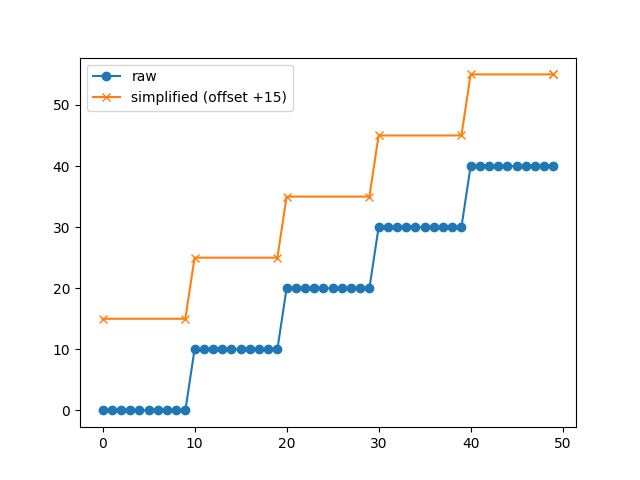# Poly_Editor - add draggable markers individually on polygon (or plot it with less markers)

I’m using matplotlib Poly_Editor to create an editable contour, but for the purpose of my project it would be interesting to plot de curve with fewer draggable points/markers. Or (it would be even better) plot it without any markers and let de user add it if they wish.

I’m using this code from Matplotlib examples, along with some more funcionalities that don’t affect this issue, and the image I’m getting (zoomed in) is the one bellow.

I tried the parameter “markevery” from Line2D but it doesn’t work for my purpose and ends up messing the lines when you drag de markers.

``````self.line = Line2D(x, y, color='b', marker='o', markerfacecolor='r', linewidth=1, markevery=10
fillstyle=None, solid_capstyle='butt', solid_joinstyle='round', animated=True)
``````

I know the markers are added in all the points of the polygon, and because mine have a lot of points, there will be a lot of markers.

What it thought so far (even though I couldn’t implement my ideas):

• something similar to the adding/deleting points function from the Poly_Editor code;
• plot the steady line of the polygon and if the user wish to edit it the editable line with markers would appear with half of the points of the original polygon array (not ideal) ;

If someone could help it would be great, I’m struggling with this for days.It looks like you have a bunch of runs of approximately straight lines which could be simplified to a much smaller number of points. Once you have done that I think the “stock” interaction will work much better.

To do the simplification you can probably use tools in mpl via `Path.iter_segments` (sorry, I do not have time to write up a worked example) or a home-grown implementation (basically ask if for any three sequential points on your polygon, is the middle one co-linear with the other two, if so drop it. repeat until you have no extra points).

@tacaswell Thanks for the ideas!
The only way I’m comming up with to check if the 3 points are collinear is with a while and 3 nested for loops, wich is a bad idea. Do you know if there’s a smarter and simpler way to do this?

And thanks again, this already helped me a lot

I think you can use matplotlib.path — Matplotlib 3.5.3 documentation which is what we use internally for simplifying paths (mostly for performance issues). Due to the way that we use it internally (on-the-fly during drawing) the API is not quite what you want, but the following shows how it works:

``````import numpy as np
from matplotlib.path import Path
import matplotlib as mpl

N = 10
M = 50

x = np.arange(M)
y = np.zeros_like(x)

for j in range(0, int(M / N)):
y[j * N : (j + 1) * N] = j * 10

p = Path(np.vstack([x, y]).T)
new_pts = []
for (v, code) in p.iter_segments(simplify=True):
new_pts.append(v)

x_trim, y_trim = np.vstack(new_pts).T

fig, ax = plt.subplots()

ax.plot(x, y, marker="o", label="raw")

ax.plot(x_trim, y_trim + 15, marker="x", label="simplified (offset +15)")
ax.legend()
plt.show()

``````I think you can wrap this up an a function that is better tuned to what you need. There is a `Path` object that underlies the polygon object so you may be able to extract and use that.

As a side note, they way we implement it is a single pass iterative algorithm that is roughly [Warning this almost certainly does not work as written and has the edge cases wrong]

``````
def simplify(raw_generator):
last_point = next(raw_generator)
yield last_point
cur_point = next(raw_generator)
for next_point in raw_generator:
if co_linear(last_point, cur_point, next_point):
cur_point = next_point
else:
yield cur_point
last_point = cur_point
cur_point = next_point
yield next_point
``````

The actual implementation in in c++ at matplotlib/path_converters.h at 5c4595267ccd3daf78f5fd05693b7ecbcd575c1e · matplotlib/matplotlib · GitHub so this has dropped lots of details!

1 Like

@tacaswell The iter method worked perfectly, huge thank you!